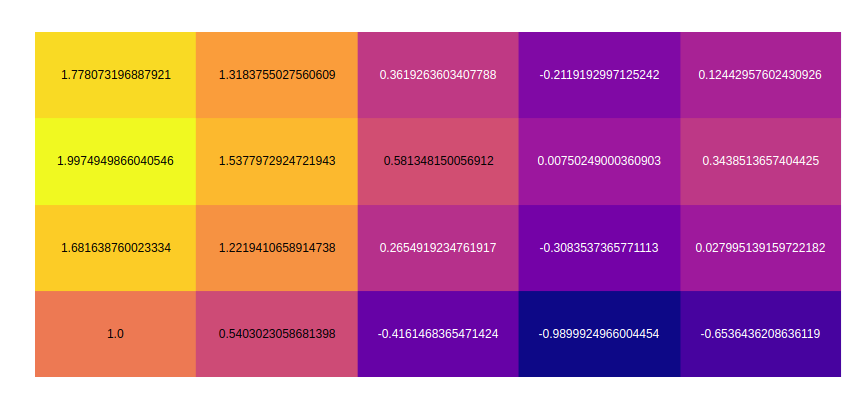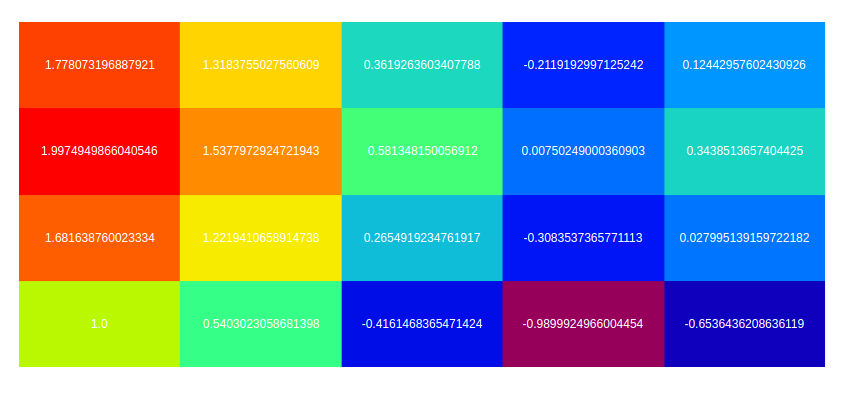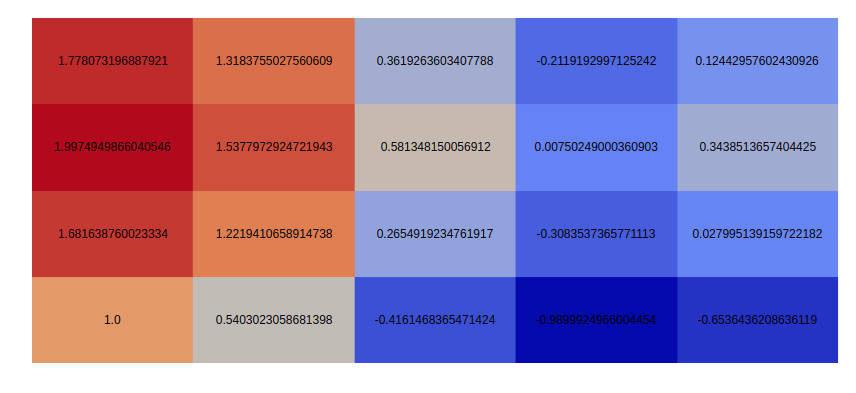GeeksforGeeks App
Open AppBrowser
Continue

# Annotated Heatmaps using Plotly in Python

A Plotly is a Python library that is used to design graphs, especially interactive graphs. It can plot various graphs and charts like histogram, barplot, boxplot, spreadplot, and many more. It is mainly used in data analysis as well as financial analysis. plotly is an interactive visualization library.

## Annotated Heatmaps

Annotated Heatmaps is the most important component of the heatmap as they shows additional information that associates with rows or columns in the heatmap. Annotation heatmaps will be represented as rows of grids through which multiple metrics can be compared to others.

Syntax: create_annotated_heatmap(z, x=None, y=None, annotation_text=None, colorscale=’Plasma’, font_colors=None, showscale=False, reversescale=False)

Parameters:

x: x axis labels.

y: y axis labels.

z:  z matrix to create heatmap.

annotation_text: Text strings for annotations. Should have the same dimensions as the z matrix. If no text is added, the values of the z matrix are annotated. Default = z matrix values.

colorscale: heatmap colorscale.

Example:

## Python3

 `import` `plotly.figure_factory as ff``import` `numpy as np`` ` `feature_x ``=` `np.arange(``0``, ``10``, ``2``)``feature_y ``=` `np.arange(``0``, ``10``, ``3``)`` ` `# Creating 2-D grid of features``[X, Y] ``=` `np.meshgrid(feature_x, feature_y)`` ` `Z ``=` `np.cos(X ``/` `2``) ``+` `np.sin(Y ``/` `4``)`` ` `fig ``=` `ff.create_annotated_heatmap(Z)``fig.show()`

Output:## Defining Colorscale

In plotly, colorscale or color chart is a flat physical object which have different types of colors. It can be set in this graph using the colorscale parameter.

Example 1:

## Python3

 `import` `plotly.figure_factory as ff``import` `numpy as np`` ` `feature_x ``=` `np.arange(``0``, ``10``, ``2``)``feature_y ``=` `np.arange(``0``, ``10``, ``3``)`` ` `# Creating 2-D grid of features``[X, Y] ``=` `np.meshgrid(feature_x, feature_y)`` ` `Z ``=` `np.cos(X ``/` `2``) ``+` `np.sin(Y ``/` `4``)`` ` `fig ``=` `ff.create_annotated_heatmap(Z, colorscale``=``'rainbow'``)``fig.show()`

Output:`import` `plotly.figure_factory as ff``import` `numpy as np`` ` `feature_x ``=` `np.arange(``0``, ``10``, ``2``)``feature_y ``=` `np.arange(``0``, ``10``, ``3``)`` ` `# Creating 2-D grid of features``[X, Y] ``=` `np.meshgrid(feature_x, feature_y)`` ` `Z ``=` `np.cos(X ``/` `2``) ``+` `np.sin(Y ``/` `4``)`` ` `custom ``=` `[[``0``, ``'green'``], [``0.5``, ``'red'``]]`` ` `fig ``=` `ff.create_annotated_heatmap(Z, colorscale``=``custom)``fig.show()`